# Polynomial Transformation

## Producer Field Guide

HGD_Product
Producer Field Guide
HGD_Portfolio_Suite
Producer

Polynomial equations are used to convert source file coordinates to rectified map coordinates. Depending upon the distortion in the imagery, the number of GCPs used, and their locations relative to one another, complex polynomial equations may be required to express the needed transformation. The degree of complexity of the polynomial is expressed as the order of the polynomial. The order is simply the highest exponent used in the polynomial.

The order of transformation is the order of the polynomial used in the transformation. ERDAS IMAGINE allows 1st- through nth-order transformations. Usually, 1st-order or 2nd-order transformations are used.

You can specify the order of the transformation you want to use in the Transform Editor.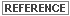A discussion of polynomials and order is included in Math Topics.

Transformation Matrix

A transformation matrix is computed from the GCPs. The matrix consists of coefficients that are used in polynomial equations to convert the coordinates. The size of the matrix depends upon the order of transformation. The goal in calculating the coefficients of the transformation matrix is to derive the polynomial equations for which there is the least possible amount of error when they are used to transform the reference coordinates of the GCPs into the source coordinates. It is not always possible to derive coefficients that produce no error. For example, in the figure below, GCPs are plotted on a graph and compared to the curve that is expressed by a polynomial.

Polynomial Curve compared to GCPs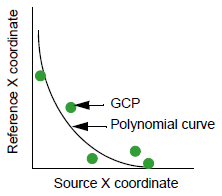Every GCP influences the coefficients, even if there is not a perfect fit of each GCP to the polynomial that the coefficients represent. The distance between the GCP reference coordinate and the curve is called RMS error, which is discussed later in this chapter. The least squares regression method is used to calculate the transformation matrix from the GCPs. This common method is discussed in Statistics textbooks.

Linear Transformations

A 1st-order transformation is a linear transformation. It can change:

• location in X or Y
• scale in X or Y
• skew in X or Y
• rotation

First-order transformations can be used to project raw imagery to a planar map projection, convert a planar map projection to another planar map projection, and when rectifying relatively small image areas. You can perform simple linear transformations to an image displayed in a Viewer or to the transformation matrix itself. Linear transformations may be required before collecting GCPs on the displayed image. You can reorient skewed Landsat TM data, rotate scanned quad sheets according to the angle of declination stated in the legend, and rotate descending data so that north is up.

A 1st-order transformation can also be used for data that are already projected onto a plane. For example, SPOT and Landsat Level 1B data are already transformed to a plane, but may not be rectified to the desired map projection. When doing this type of rectification, it is not advisable to increase the order of transformation if at first a high RMS error occurs. Examine other factors first, such as the GCP source and distribution, and look for systematic errors.

ERDAS IMAGINE provides the following options for 1st-order transformations:

• scale
• offset
• rotate
• reflect

Scale

Scale is the same as the zoom option in the Viewer, except that you can specify different scaling factors for X and Y.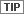If you are scaling an image in the Viewer, the zoom option undoes any changes to the scale that you do, and vice versa.

Offset

Offset moves the image by a user-specified number of pixels in the X and Y directions. For rotation, you can specify any positive or negative number of degrees for clockwise and counterclockwise rotation. Rotation occurs around the center pixel of the image.

Reflection

Reflection options include the following operations:

• left to right reflection
• top to bottom reflection
• left to right and top to bottom reflection (equal to a 180° rotation)

Linear adjustments are available from the Viewer or from Transform Editor. You can perform linear transformations in the Viewer and then load that transformation to Transform Editor, or you can perform the linear transformations directly on the transformation matrix.

The figure below illustrates how the data are changed in linear transformations.

Linear Transformations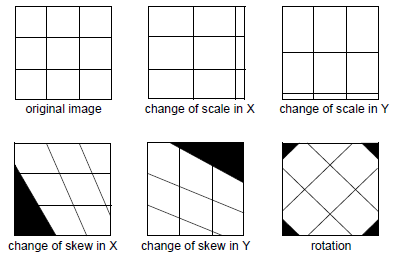The transformation matrix for a 1st-order transformation consists of six coefficients—three for each coordinate (X and Y).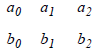Coefficients are used in a 1st-order polynomial as follows: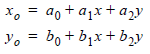Where:

x and y are source coordinates (input)

xo and yo are rectified coordinates (output)

The coefficients of the transformation matrix are as above.The position of coefficients in the matrix and the assignment of the coefficients in the polynomial is an ERDAS IMAGINE convention. Other representations of 1st-order transformation matrix may take a different form.

Nonlinear Transformations

Transformations of the 2nd-order or higher are nonlinear transformations. These transformations can correct nonlinear distortions. The process of correcting nonlinear distortions is also known as rubber sheeting. The figure below illustrates the effects of some nonlinear transformations.

Nonlinear Transformations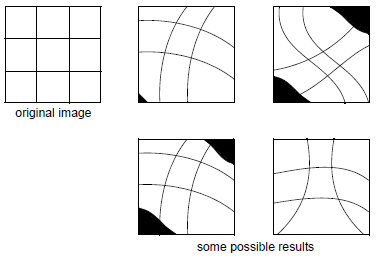Second-order transformations can be used to convert Latitude and Longitude data to a planar projection, for data covering a large area (to account for the Earth’s curvature), and with distorted data (for example, due to camera lens distortion). Third-order transformations are used with distorted aerial photographs, on scans of warped maps and with radar imagery. Fourth-order transformations can be used on very distorted aerial photographs.

The transformation matrix for a transformation of order t contains this number of coefficients: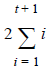It is multiplied by two for the two sets of coefficients—one set for X, one for Y.

An easier way to arrive at the same number is: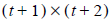Clearly, the size of the transformation matrix increases with the order of the transformation.

Higher Order Polynomials

The polynomial equations for a t-order transformation take this form: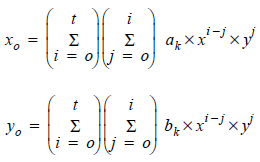Where:

t = order of the polynomial

ak and bk = coefficients

Subscript k is determined by: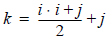An example of 3rd-order transformation equations for X and Y, using numbers, is: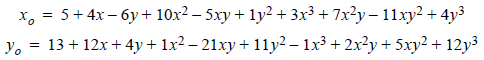These equations use a total of 20 coefficients, or

(3 + 1) x (3 + 2)

Effects of Order

The computation and output of a higher-order polynomial equation are more complex than that of a lower-order polynomial equation. Therefore, higher-order polynomials are used to perform more complicated image rectifications. To understand the effects of different orders of transformation in image rectification, it is helpful to see the output of various orders of polynomials.

The following example uses only one coordinate (X), instead of two (X,Y), which are used in the polynomials for rectification. This enables you to draw two-dimensional graphs that illustrate the way that higher orders of transformation affect the output image.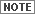Because only the X coordinate is used in these examples, the number of GCPs used is less than the number required to actually perform the different orders of transformation.

Coefficients like those presented in this example would generally be calculated by the least squares regression method. Suppose GCPs are entered with these X coordinates:

 Source X Coordinate(input) Reference X Coordinate(output) 1 17 2 9 3 1

These GCPs allow a 1st-order transformation of the X coordinates, which is satisfied by this equation (the coefficients are in parentheses):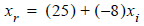Where:

xr = reference X coordinate

xi = source X coordinate

This equation takes on the same format as the equation of a line (y = mx + b). In mathematical terms, a 1st-order polynomial is linear. Therefore, a 1st-order transformation is also known as a linear transformation. This equation is graphed in the figure below.

Transformation Example—1st-Order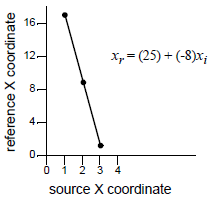However, what if the second GCP were changed as follows?

 Source X Coordinate(input) Reference X Coordinate(output) 1 17 2 7 3 1

These points are plotted against each other in the figure below.

Transformation Example—2nd GCP Changed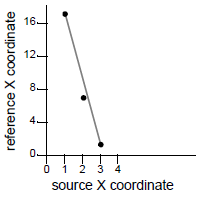A line cannot connect these points, which illustrates that they cannot be expressed by a 1st-order polynomial, like the one above. In this case, a 2nd-order polynomial equation expresses these points: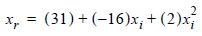Polynomials of the 2nd-order or higher are nonlinear. The graph of this curve is drawn in the figure below.

Transformation Example—2nd-Order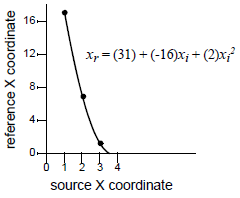What if one more GCP were added to the list?

 Source X Coordinate(input) Reference X Coordinate(output) 1 17 2 7 3 1 4 5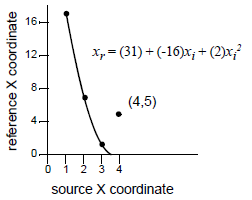As illustrated in the figure above, this fourth GCP does not fit on the curve of the 2nd-order polynomial equation. To ensure that all of the GCPs fit, the order of the transformation could be increased to 3rd-order. The equation and graph in the figure below could then result.

Transformation Example—3rd-Order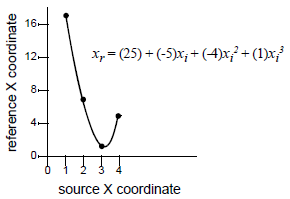The figure above illustrates a 3rd-order transformation. However, this equation may be unnecessarily complex. Performing a coordinate transformation with this equation may cause unwanted distortions in the output image for the sake of a perfect fit for all the GCPs. In this example, a 3rd-order transformation probably would be too high, because the output pixels would be arranged in a different order than the input pixels, in the X direction.

 Source X Coordinate(input) Reference X Coordinate(output) 1 xo(1) = 17 2 xo(2) = 7 3 xo(3) = 1 4 xo(4) = 5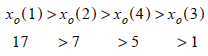Transformation Example—Effect of a 3rd-Order Transformation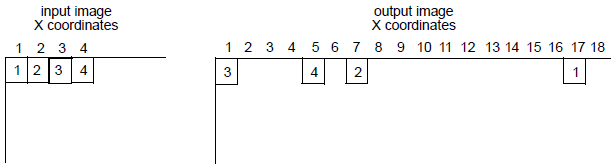In this case, a higher order of transformation would probably not produce the desired results.

Minimum Number of GCPs

Higher orders of transformation can be used to correct more complicated types of distortion. However, to use a higher order of transformation, more GCPs are needed. For instance, three points define a plane. Therefore, to perform a 1st-order transformation, which is expressed by the equation of a plane, at least three GCPs are needed. Similarly, the equation used in a 2nd-order transformation is the equation of a paraboloid. Six points are required to define a paraboloid. Therefore, at least six GCPs are required to perform a 2nd-order transformation. The minimum number of points required to perform a transformation of order t equals: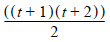Use more than the minimum number of GCPs whenever possible. Although it is possible to get a perfect fit, it is rare, no matter how many GCPs are used.

For 1st- through 10th-order transformations, the minimum number of GCPs required to perform a transformation is listed in the following table:

 Order ofTransformation Minimum GCPsRequired 1 3 2 6 3 10 4 15 5 21 6 28 7 36 8 45 9 55 10 66For the best rectification results, you should always use more than the minimum number of GCPs, and they should be well-distributed.